# Finding the Channels

Last winter, Aktham asked on MATLAB Answers how to find the channels in this image.
imshow(A)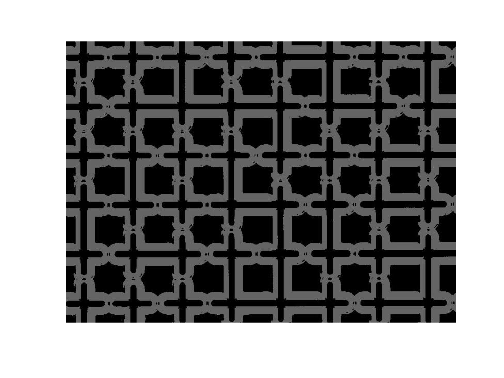Image credit: Aktham Shoukry. Used with permission.
Here is an example of what Aktham meant by "channel."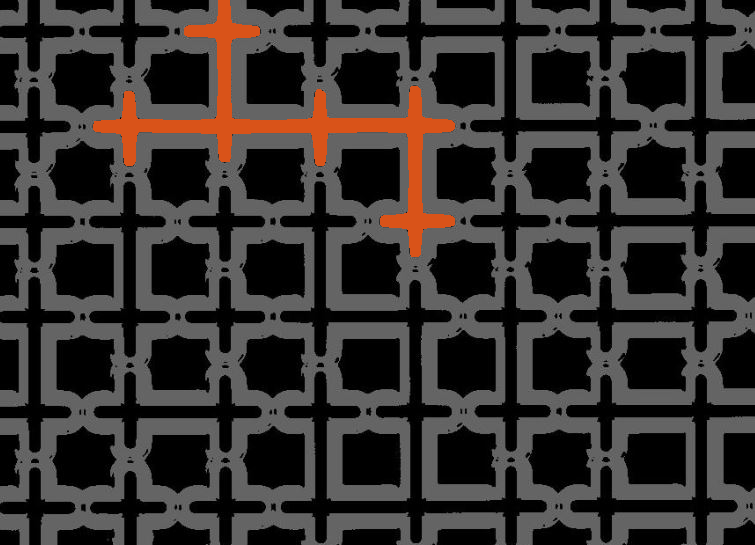This post shows one way to accomplish the task, using erosion, morphological reconstruction, and some image arithmetic.
The channels are relatively thin in one dimension, either horizontally or vertically. So, a morphological erosion can be constructed that will keep a portion of each grain, while completely eliminating the channels.
First, a bit of preprocessing.
Ag = im2gray(A);
B = imcomplement(Ag);
imshow(B)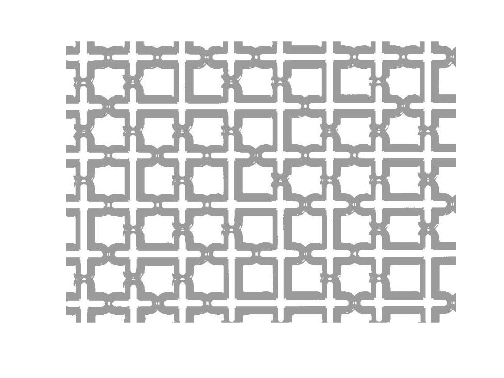The channels are about 10 pixels thick, while the square-ish "grains" are about 50-by-50. Let's erode with a square structuring element with a size that's in between.
C = imerode(B,ones(25,25));
imshow(C)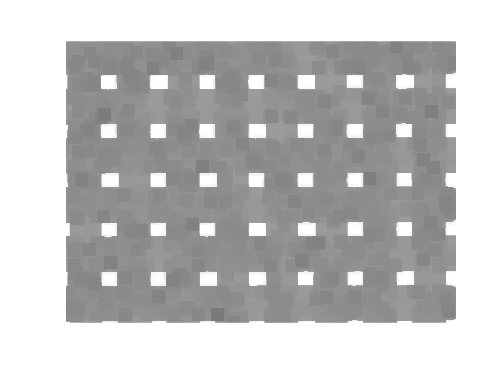Next, use morphological reconstruction to restore the original grain shapes.
D = imreconstruct(C,B);
imshow(D)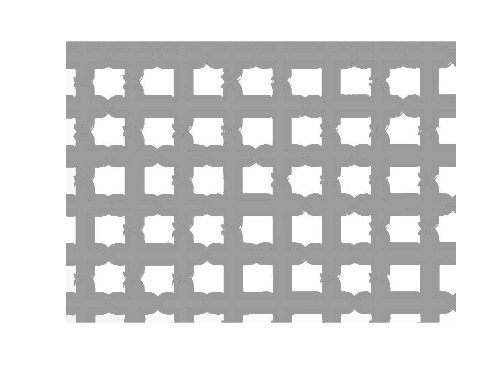Complement the image to make the grains dark again.
E = imcomplement(D);
imshow(E)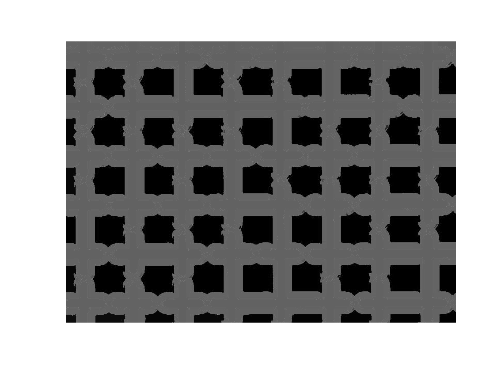Subtracting the original grayscale image from E gets us very close.
F = E - Ag;
imshow(F)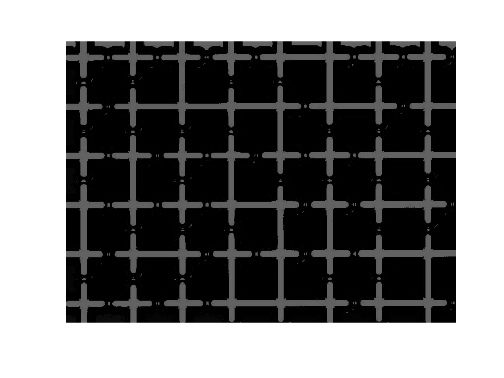G = imbinarize(F);
imshow(G)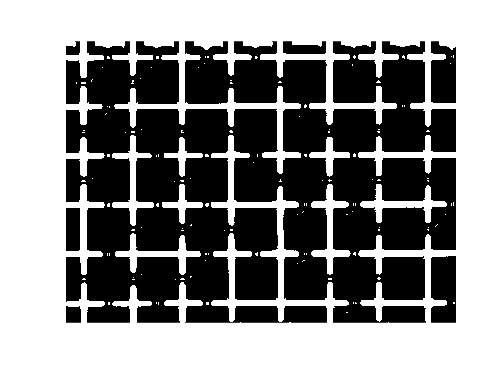A final cleanup of the small objects, using either bwareafilt or bwareaopen, gives us our result.
H = bwareaopen(G,50);
imshow(H)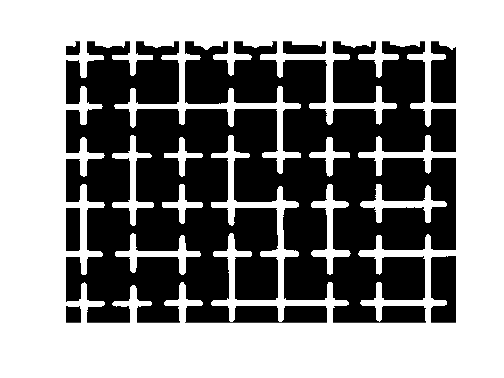The function imoverlay gives us a nice visualization of the result. (You could also use imfuse.)
K = imoverlay(Ag,H,[.8500 .3250 .0980]);
imshow(K)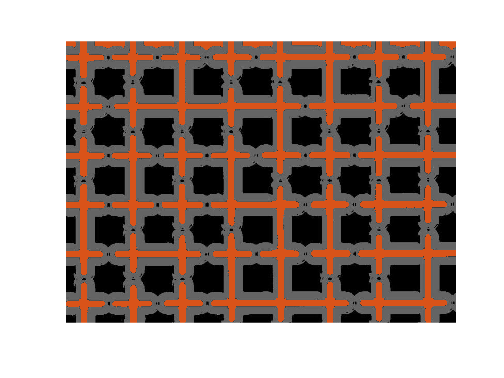Note, however, that our result isn't quite perfect. There are partially cropped-off grains along the top border that have been identified as channels. Normally, I would recommend using imclearborder as a preprocessing step, but in this case, imclearborder eliminates too many of the desired channels.